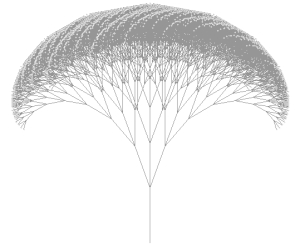### Broccoli (Recursion)

10 points

Recursion is a powerful technique in programming that involves solving a problem by dividing the problem into small pieces, and then putting the pieces back together. In recursion, you typically use the 'recursive case' until you've finished dividing the problem into small pieces. At this point, the 'base case' in recursion provides a stopping point. Then you put the small pieces together and generate a solution.

Factorial
A good example of a simple use of recursion is to calculate n!, where n is an integer. You can calculate n! = (n)(n-1)(n-2)...(3)(2)(1), of course. A recursive approach is: 'if n is 1, the value is 1. If n is any number larger than 1, find the factorial by multiplying n by (n-1)!.' The 'if n is 1, the value is 1' part is the 'base case'. The 'multiply n by (n-1)' part is the 'recursive case'.

Let's take 4! as an example. How do you find 4! recursively? Since 4 is larger than 1, we calculate: (4)(3!). How do we find (3!)?. It equals (3)(2!). How do we find (2!)? It equals (2)(1!). How do we find (1!)?. We have arrived at the base case, and we know that 1! equals 1. Working backwards then, 2! = (2)(1) = 2. 3! = (3)(2) = 6. 4! = (4)(6) = 24. So after breaking the problem of finding 4! down into smaller and smaller factorial problems, we put the pieces back together (by multiplying) and find that 4! = 24.

BroccoliHere is a computer-generated image that is supposed to resemble broccoli. This broccoli is the result of applying a simple recursion. The 'recursive case' says: to draw broccoli, first draw a stem. Then, starting at the end of the stem, draw a new smaller stem, then rotate left 20° and draw a new smaller stem, then rotate right 20° and draw a third new smaller stem. Each of these three new stems will continue this process. (Each time a new stem is drawn, it is only 80% of the size of the 'parent' stem.) This recursive process continues, with each stem 'parenting' three more stems, until the 'base case' is reached. The 'base case' says: when the stem of the broccoli is below a certain length, instead of drawing three more stems, draw a bud, and stop. This way you should get a full head of broccoli, topped by buds or flowers, and the process has a way to stop.

You can download below the 4 Java source files you'll need. The description of what you'll need to write is inside each Java file.

Starting Point

You can download Stem.java, Flower.java, Broccoli.java and Board.java to use as a starting point in writing your program.

Note: This example is taken directly from Java: An eventful approach by Bruce, Danyluk, and Murtagh.